Sample Module

Calculus II

Introduction

The integral plays a very important role in almost all engineering and physics disciplines. Business and economics also have a role for integration. We don't delve into that role in this module, limiting our discussion and examples to the purely mathematical. As you learned in the prior module, the derivative yields the slope of a curve at a particular point. The integral is the opposite of the derivative, and simple integrals are known as anti-derivatives. Such simple integrals yield the area under a curve and above the x-axis between two points, a and b (with b > a).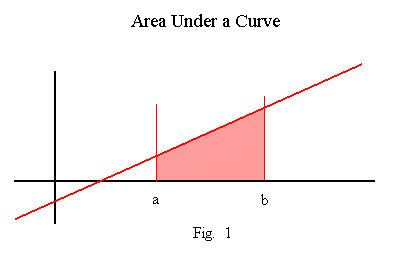Area - Case 1

In the simplest case, the curve, the area under which we wish to find, is a straight horizontal line. The function, f(x), is a constant expressed as (say) f(x) = k, where k > 0. The graph of f(x) = k above the x-axis and between points a and b, (b > a), is shown below.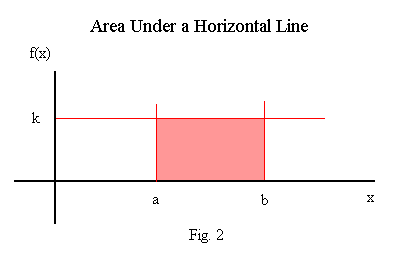As you know, the area of a rectangle is its height times its width. In this case, the height of the rectangle is k and its width is (b-a). This yields an area of A = k * (b-a). Finding the area of this rectangle by integration should yield the identical result.

A = ∫ab   k dx = k*x |ab   = k*b - k*a => k * (b-a). Q.E.D.

Area - Case 2

In the next simplest case, the curve, the area under which we wish to find, is a straight upward-sloping line through the origin. This function is expressed as (say) f(x) = x. The graph of f(x) = x above the x-axis and between zero and point b, (b > 0), is shown below.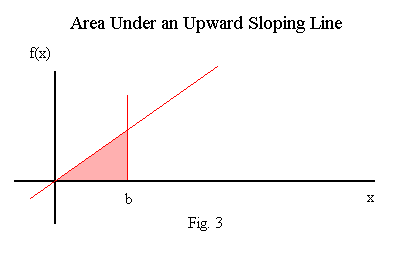As you know, the area of a triangle is one half its height times its base. In this case, the height of the rectangle is f(b) = b and its base is b, (b-0). This yields an area of A = 0.5 * b2. Finding the area of this rectangle by integration should yield the identical result.

A = ∫ab   x dx = 0.5 * x2 |0b   = 0.5 * b2 - 0.5 * 02 => 0.5 * b2. Q.E.D.

Area - Case 3

A slightly more complex case is that of trapezoid areas. These would be created by functions of the form f(x) = x where the lower limit is not 0. Say f(x) = 2 * x and the limits are a and b, (b > a > 0). Its graph is shown below. To compute the area of a trapezoid, we must divide the area into 2 parts; an upper triangle and a lower rectangle. This case combines the techniques demonstrated in two prior cases.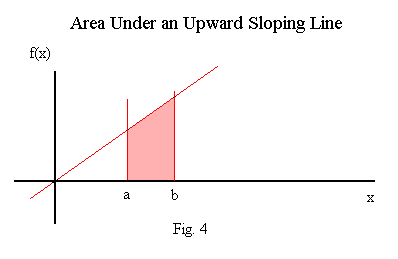Say the function is f(x) = 2 * x. For the triangle, the base is b - a and the height is f(b) (= 2*b) - f(a) (= 2*a). This yields and area for the triangle of At = 0.5 * (b - a) * (2b - 2a) = (b - a)2. For the rectangle, the height is f(a) (= 2*a) and the width is b - a. This yields an area for the rectangle of Ar = 2 * a * (b - a). The total area for the trapezoid is At + Ar = (b - a)2 + 2 * a * (b - a). This expression factors to A = b2 - a2.

Finding the area of this trapezoid by integration should yield the identical result. The integral of the area is as follows.

A = ∫ab   2*x dx = x2 |ab   = b2 - a2. Q.E.D.

Area - Case 4

The value of using integration to compute area is seen when dealing with functions tht are not straight lines, such as f(x) = x2. This is because non-linear functions cannot easily be broken into geometric shapes whose areas are easy to compute. Consider the function f(x) = x2. The area below that curve and above the x-axis between the points 1 and 4 is shown in the graph below.The area in question is expressed by the integral A = ∫14   x2 dx = 0.333 * x3 |14   => 0.333 * [43 - 13] = 21.

Area - Case 5

When the function is symmetric about a vertical axis, it is possible to compute half the area and multiply by 2. The parabola f(x) = x2 is symmetric about the vertical axis at x = 0. If we want to find the area under f(x) = x2 and above the x-axis on the interval [-a, a], we can find the area on the interval [0, a] and double it. Computing the area both ways will confirm this.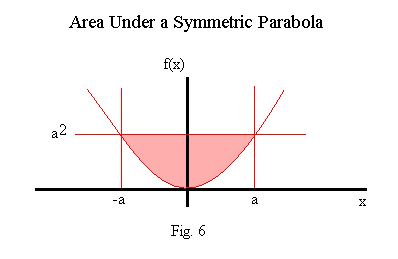Say we set the value of a in figure 6 above to 3. The area under the curve f(x) = x2 and above the x-axis on the interval [-3, 3] is expressed as

A = ∫-33   x2 dx = 1/3 * x3 |-33   = 1/3 * [33 - (3)3] = 1/3 * [27 - (-27)] = 18.0.

Expressing the integral as twice the area under the curve and above the x-axis on the interval [0,3] yields the integral

A = 2 * ∫03   x2 dx = 2 * 1/3 * x3 |03   = 12 * /3 * [33 - 0] = 2 * 1/3 * 27 = 18.0. Q.E.D.

Area - Case 6

When part of a function's curve falls below the x-axis, so that the area being found is above the curve and below the x-axis, the area in question will have a negative value. Area is not a construct that has meaning when negative. Area, like length or width, must be positive. Though for some complex problems negative "area" will have meaning, for this module area cannot be negative.

The procedure to find the area under a curve that falls below the x-axis involves first finding the point at which the curve intersects the x-axis. On the interval [a,b] with f(a) < 0 and f(b) > 0, we must find the point c at which f(c) = 0. The we compute the areas of the two intervals, [a,c] and [c,b], separately. However, wetake the absolute value of the area that is below the x-axis. So the total area will equal the sum of the two sub-areas. Let Aa be the area for the interval [a,c] and Ab for the interval [c,b], then A = |Aa| + Ab.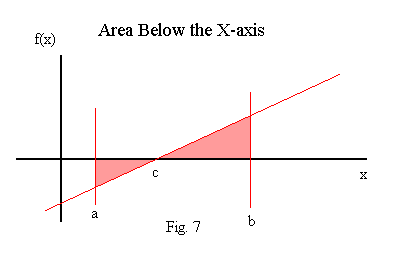Say f(x) = 2*x - 4 and the interval is [0,8]. We find the point, c, in figure 7 by setting f(x) equal to zero: 2*x - 4 = 0 => x = 2. The area on [0,8] is

A = |∫02   (2*x - 4) dx| + ∫28   (2*x - 4) dx = |(x2 - 4*x) |02  | + (x2 - 4*x) |28   =>

A = |[(22 - 4*2) - (02 - 4*0)]| + [(82 - 4*8) - (22 - 4*2)] = |4 - 8| + [(64 - 32) - (4 - 8)] = 40.0.

Failing to take the absolute value of the area below the x-axis will yield the incorrect area. The integral would be

A = ∫08   (2*x - 4) dx = (x2 - 4*x) |08   = 64 - 32 = 32.

Area - Case 7

The examples above show the area between the curve of a function and the x-axis, the line at which f(x) = 0. We now consider finding the area between two different functions, say f(x) and g(x). The area between the two functions on the interval [a,b] is expressed as A = ∫ab   [f(x) - g(x)] dx. Note that when g(x) = 0, we have the previously demonstrated scenarios.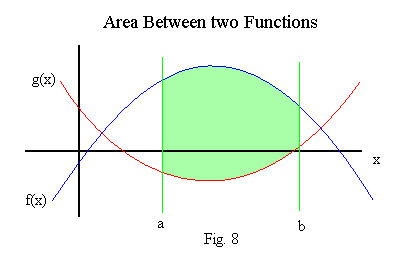Say f(x) = √x, g(x) = -1.0, and the interval is [1,4]. The appropriate integral is

A = ∫14   [√x - (-1)] dx.

This yields

A = [2/3 * x3/2 - (-x)] |14   = [2/3 * 43/2 - (-4)] - [2/3 * 1 - (-1)] = (16/3 + 4) - (2/3 + 1) = 23/3 or 7 2/3.

A more complex example is f(x) = -x2 + 4, g(x) = 1/2 * x2 - 2, and the interval is [-1,1]. The appropriate integral is

A = ∫-11   [(-x2 + 4) - (1/2 * x2 - 2)] dx => ∫-11   (-5/2 x2 + 6) dx.

This yields

A = (1/3 * (-5/2) * x3 + 6 * x) |-11   = (-5/6 + 6) - (5/6 - 6) = -10/6 + 12 = 31/3 or 10 1/3.

Area - Case 8

When the two functions intersect within the limits of integration then finding the area must be performed in 2 steps. First the point of intersection is found, and second the absolute values of the areas of each section are computed. This is same as the procedure demonstrated in case 6 for a function that falls below the x-axis.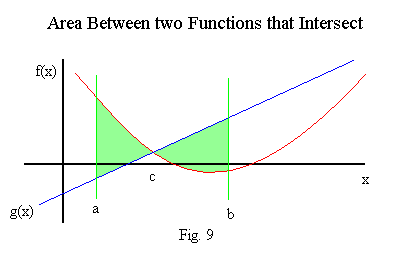Say f(x) = x - 2, g(x) = (x - 4)2, and the interval is [2,6]. We find the point, c, in figure 9 by setting f(x) equal to g(x): x - 2 = (x - 4)2 => x2 - 9 8 x + 18 = 0. This quadratic equation has the roots 3 and 6; (x - 3) * (x - 6) = 0. The two curves intersect at x = 3 and x = 6. Since x = 3 is in the interval, the two sub intervals are [2,3] and [3,6]. The area is

A = |∫23   [(x - 2) - (x - 4)2] dx| + ∫36   [(x - 2) - (x - 4)2] dx   =>   A = |∫23   (-x2 + 9 * x - 18) dx| + ∫36   (-x2 + 9 * x - 18) dx.

The result is

A = |[-1/3 * x3 + 9/2 * x2 - 18 * x]|23   | + [-1/3 * x3 + 9/2 * x2 - 18 * x]|36

= |(-1/3 * 33 + 9/2 * 32 - 18 * 3) - (-1/3 * 23 + 9/2 * 22 - 18 * 2)| + [(-1/3 * 63 + 9/2 * 62 - 18 * 6) - (-1/3 * 33 + 9/2 * 32       - 18 * 3)]

= |-22 1/2 - (-20 2/3)| + 18 - (-22 1/2) = |1 5/6| + 4 1/2 = 6 1/3.

Sample Questions about the Area under a Curve.

Motion and Position of an Object

Recall from the module on differentiation that the derivative of the position function with respect to time yields tehe velocity function, and the derivative of the velocity function with respect to time yields the acceleration function. By applying the anti-derivative principle, we obtain the velocity function by integrating the acceleration function with respect to time, and the position function by integrating the velocity function with respect to time.

The acceleration and velocity integrals are indefinite. The definite integral of the velocity function yields the distance travelled within the time span denoted by the integral limits. Determining position and distance travelled through integration has important engineering applications.

Velocity

We start with examples that find velocity.

Example 1.

A ball is dropped from the roof of buiding that is 50 meters high. How fast is the ball falling 2 seconds after its release?

Note that the acceleration due to gravity is -9.8 meters per second squared (m/s2), with the minus sign meaning a downward direction. The acceleration function is a(t) = -9.8. The velocity function is the indefinite integral of the acceleration function,

v(t) = ∫-9.8 dt = -9.8 * t + C.

We now need to determine the value of the constant, C. The initial velocity is 0.0, so v(0) = 0. Therefore, -9.8 * 0 + C = 0 => C = 0.0 The full velocity function is v(t) = -9.8 * t. At t = 2,

v(2) = -9.8 * 2 = -19.8.

The ball is falling at a speed of 19.8 meters per second 2 seconds after being released.

Example 2.

A ball is thrown down from the roof of buiding that is 100 meters high. Its velocity immediately after release is -5.5 m/s. How fast is the ball falling 1.5 seconds after its release?

The acceleration due to gravity is still -9.8 m/s2 so the acceleration function is again a(t) = -9.8. The velocity function is again the indefinite integral of the acceleration function,

v(t) = ∫-9.8 dt = -9.8 * t + C.

The initial velocity is -5.5, so v(0) = -5.5. Therefore, -9.8 * 0 + C = -5.5 => C = -5.5. The full velocity function is

v(t) = -9.8 * t - 5.5. At t = 1.5,

v(1.5) = -9.8 * 1.5 - 5.5 = -20.2.

The ball is falling at a speed of 20.2 m/s 1.5 seconds after being released.

Example 3.

A ball is tossed upward from the roof of buiding that is 100 meters high. Its velocity immediately after release is 8.0 m/s. How fast is the ball falling 3 seconds after its release?

The acceleration due to gravity is still -9.8 m/s2 so the acceleration function is again a(t) = -9.8. The velocity function is again the indefinite integral of the acceleration function,

v(t) = ∫-9.8 dt = -9.8 * t + C.

The initial velocity is 8.0, so v(0) = 8.0. Therefore, -9.8 * 0 + C = 8.0 => C = 8.0. The full velocity function is

v(t) = -9.8 * t + 8.0. At t = 3,

v(3) = -9.8 * 3 + 8.0 = -21.4.

The ball is falling at a speed of 21.4 m/s 3 seconds after being released.

Example 4.

Objects are subject to acceleration whenever any acts upon them, not only the force of gravity. Consider an object that can move along some coordinate line. The object is subject to a force that yields a constant acceleration of 3.5 m/s2. At a certain point on the line, when t = 0, the object's velocity is 8.5 m/s. What is the object's velocity 4 seconds later?

The acceleration function is a(t) = 3.5. The integral for the velocity function is

v(t) = ∫3.5 dt = 3.5 * t + C.

The initial velocity is 8.5, so v(0) = 8.5. Therefore, 3.5 * 0 + C = 8.5 => C = 8.5. The full velocity function is

v(t) = 3.5 * t + 8.5. At t = 4, v(4) = 3.5 * 4 + 8.5 = 22.5.

The oject is moving in the positive direction at a speed of 22.5 m/s.

Example 5.

Again consider an object that can move along some coordinate line. The object is subject to a force that yields a variable acceleration of 2 * t - 4 m/s2. At a certain point on the line, when t = 0, the object's velocity is -2 m/s. What is the object's velocity 5 seconds later?

The acceleration function is a(t) = 2 * t - 4. The integral for the velocity function is

v(t) = ∫2 * t - 4 dt = t2 - 4 * t + C.

The initial velocity is -2, so v(0) = -2. Therefore, 02 - 4 * 0 + C = -2 => C = -2. The full velocity function is

v(t) = t2 - 4 * t - 2. At t = 5,

v(5) = 52 - 4 * 5 - 2 = 3.0.

The oject is moving in the positive direction at a speed of 3.0 m/s.

Position and Distance

We continue with examples that find position and distance.

Example 1.

A ball is dropped from the roof of buiding that is 50 meters high. How far is the ball from the ground 2 seconds after its release?

The acceleration function is a(t) = -9.8. The velocity function, from the corresponding example above, is

v(t) = -9.8 * t.

The position function is the indefinite integral of the velocity function;

p(t) = ∫-9.8 * t dt = -4.9 * t2 + C.

To determine the value of the constant, C, we need to find out the position of the ball at time zero. That is given as 50 meters above the ground. The full position function is therefore

p(t) = -4.9 * t2 + 50.

The position of the ball after 2 seconds is given by

p(2) = -4.9 * 22 + 50 = 30.4.

The ball is 30.4 meters above the ground after falling for 2 seconds.

The distance travelled by the ball during the 2 seconds of falling is expressed by the definite velocity integral evaluated over the 2 seconds. The expression is

d = ∫02   -9.8 * t dt = -4.9 * t2 |02   = -4.9 * 4 - 0 = 19.6.

The ball travelled 19.6 meters during its 2 seconds of falling.

Example 2.

A ball is tossed upward from the roof of buiding that is 100 meters high. Its velocity immediately after release is 8.0 m/s. How far is the ball from the ground 3 seconds after its release? And how far does the ball travel during the fourth second of its fall?

The acceleration function is a(t) = -9.8. The velocity function, from the corresponding example above, is

v(t) = -9.8 * t + 8.

The position function is the indefinite integral of the velocity function;

p(t) = ∫-9.8 * t + 8 dt = -4.9 * t2 + 8 * t + C.

To determine the value of the constant, C, we need the position of the ball at time zero; 100 meters above the ground. The full position function is therefore

p(t) = -4.9 * t2 + 8 * t + 100.

The position of the ball after 3 seconds is given by

p(3) = -4.9 * 32 + 8 * 3 + 100 = 79.9.

The ball is 79.9 meters above the ground 3 seconds after its release.

The distance travelled by the ball during the fourth second of falling is expressed by the definite velocity integral evaluated over the 1 second. The expression is

d = ∫34   -9.8 * t + 8 dt

= -4.9 * t2 + 8 * t |34

= (-4.9 * 42 + 8 * 4) - (-4.9 * 32 + 8 * 3) = -46.4 - (-20.1) = -26.3.

The ball travelled (downward) 26.3 meters during the fourth second after its release.

Example 3.

An object that can move along a coordinate line is subject to a force that yields a constant acceleration of 3.5 m/s2. At a certain point on the line, when t = 0, the object's velocity is 8.5 m/s and its position is -22.4 meters. What is the object's position 4 seconds later? What distance does the object travel from t = 6 to t = 8?

The acceleration function is a(t) = 3.5. The velocity function is

v(t) = 3.5 * t + 8.5.

The position function is

p(t) = ∫3.5 * t + 8.5 dt = 1.75 * t2 + 8.5 * t + C.

The initial position is -22.5, so 1.75 * 0 + 8.5 * 0 + C = -22.4 => C = -22.4. The full position function is

p(t) = 1.75 * t2 + 8.5 * t - 22.4.

At t = 4,

p(4) = 1.75 * 42 + 8.5 * 4 - 22.4 = 39.6.

The object is at point 39.6 meters at t = 4 seconds.

The distance travelled by the object from t = 6 to t = 8 is expressed by the definite velocity integral evaluated over the 2 second interval. The expression is

d = ∫68   3.5 * t + 8.5 dt

= 3.5 * t2 + 8.5 * t |68

= (1.75 * 82 + 8.5 * 8) - (3.5 * 62 + 8.5 * 6) = 180.0 - 114.0 = 66.0.

The object travelled 66.0 meters in a positive direction during the seventh and eighth seconds after its release.

Example 5.

Again consider an object that can move along some coordinate line. The object is subject to a force that yields a variable acceleration of 2 * t - 4 m/s2. At a certain point on the line, when t = 0, the object's velocity is -2 m/s and its position is 16 m. What is the object's position 6 seconds later? What distance does the object travel from t = 9 to t = 12?

The acceleration function is a(t) = 2 * t - 4. The velocity function is

v(t) = t2 - 4 * t - 2.

The position function is expressed as

∫t2 - 4 * t - 2 dt = 1/3 * t3 - 2 * t2 - 2 * t + C.

The initial position is 16 m, therefore,

p(t) = 1/3 * 03 - 2 * 02 - 2 * 0 + C = 16 => C = 16.

The full position function is

p(t) = 1/3 * t3 - 2 * t2 - 2 * t + 16.

At t = 6,

p(6) = 1/3 * 63 - 2 * 62 - 2 * 6 + 16 = 4.0.

The object is at point 4 m at time t = 6.

The distance travelled by the object from t = 9 to t = 12 is expressed by the definite velocity integral evaluated over the 3 second interval. The expression is

d = ∫912   t2 - 4 * t - 2 dt

= 1/3 * t3 - 2 * t2 - 2 * t |912

= (1/3 * 123 - 2 * 122 - 2 * 12) - (1/3 * 93 - 2 * 92 - 2 * 9) = 264.0 - 63.0 = 201.0.

The object travelled 201.0 meters in a positive direction between t = 9 and t = 12.